nextnano.com  GUI: nextnanomat  Tool: nextnano++  Tool: nextnano³  Tool: nextnano.NEGF  Download | Search | Copyright | News | Publications  * password protected nextnano³ softwareFAQ

# General

## Program window closes immediately upon finishing

Question

Unfortunately, the program window that opens after I started``` nextnano3.exe ```by double-clicking on it, closes immediately upon finishing, so I can't see the stuff that is written on the screen during program execution. Is there a way to prevent this?

Answer

Yes, there is. In Windows go to the` Start `button, select``` Run ... ```and type in` cmd `and press` Enter`.
This opens the command shell. Switch to the directory where``` nextnano3.exe ```is located by using the change directory command and type in```  nextnano3.exe ```and then press` Enter`.
You can redirect the screen output into a file by using the command: ``` nextnano3.exe > out.log```.

## doesn't work at all

Question

I get a strange message in my command prompt. Something with "keyword", "optional", "required".

Answer

You are probably trying to run a UNIX formatted ASCII file on a PC (or vice versa). Save it in PC/DOS mode or download the PC formatted input files from the download section.

## "```ERROR in OPEN file = keywords.val```"

Question

I get the following error message after I started the executable:

"```ERROR in OPEN file = keywords.val  File doesn’t exit or unit 10 is already connected!  Press any key to continue.```

Answer

The executable must lie in the same directoy as the` keywords.val`,``` database_nn3.in```,` database_nn3_keywords.val `and` input_file1.in `file if you execute the executable from the command shell.
(not true any more: CHECK: Update documentation)

### Downloads / Additional tools

Question

Where can I get the Perl script to convert output AVS/Express files (*.fld, *.dat, *.coord) into xyz files?

Answer

Follow this link to our download page: Downloads / Additional tools

### `d0`

Question

What is the meaning of` d0 `in the input file?

Answer

`d0 `or ` D0 `is a Fortran like syntax and means 100 = 1 in "double precision". You can also use` e0 `or``` E0 ```in the input file which is single-precision.
In fact, nextnano³ converts` e0 `(single precision) automatically into` d0 `(double precision) when parsing the input file.

`1d-3 `means 10-3 = 0.001
`50d-3 `means 50 * 10-3 = 0.050
`2.0d18 `means 2.0 * 1018

Meanwhile,` d0 `is optional, so intead of specifing` 0.067d0`, you can use` 0.067 `for newer versions of nextnano³.

# Materials

Where can I find a list of all materials?

Open the database file "`database_nn3.in`" with a text editor (e.g. WordPad) and search for the string "`\$default-materials`". Then you can see a list of all materials.

You can add new materials into the database very easily. Just "copy and paste" the entries of a previously defined material and modify them appropriately.

# Simulation

## Alloy composition 2D

Question

I have to simulate a 2D system where in certain regions I have alloy composition fluctuations. From experiment I have  the alloy content given in a two-dimensional grid (that is in a file with three columns: x y and alloy composition). The grid consists of 60x187 points.

Is there any simple way to input these alloy composition or do I have to define in the input file all these 60x187 numbers?

Answer

Unfortunately, it is currently not possible to read in the alloy content from a file but we are certainly planning to do so in the future.
(However, it is possible to read in a doping profile.)

You have to specify it as indicated here: `\$alloy-function`

## Band structure

Question

I am only familiar with band structures like E(kx,ky,kz), i.e. energy as a function of the k vectors in the Brillouin zone (energy dispersion). Is it true that nextnano3 plots the band structure as a function of real space (device geometry in x, y and z direction) for the minimum of the conduction and maximum of the valence bands?

Answer

Yes, it is. We are interested in the values at the Gamma point E(k=0) for the conduction band (minimum) and valence band (maxium; heavy, light and split-off hole). These energy values (which represent extrema) are plotted as a function of real space E(r) over the whole device geometry (i.e. for each material including doping effects aso.)
The same applies to the conduction band minima at the L point and X point (k0).

## Conduction/valence band plots

Question

Instead of a sharp edge in the bands at the interface of a semiconductor heterostructure from one material to the other, there is always a grid point in between with the value  0.5 * band offset. This seems wrong to me.

Answer

You are absolutely right. To save the amount of data points necessary for a 2D or 3D plot, we take the average value of the 8 points of the corresponding 8 octants (boxes) for each grid point, thus the plotted value lies in the "middle" of the real boundary values. (In 2D, average of 4 "octants".)
You can change this default assignement by including in your input file the keyword``` \$output-file-format```. If you make your grid denser at the heterointerface, you can also modify the appearance of the resulting plot.

``` !--------------------------------------------------!  \$output-file-format                                !   simulation-dimension = 3                          ! 2D or 3D   file-format          = AVS-ASCII                  !   resolution           = all                        ! all/average  \$end_output-file-format                            !  !--------------------------------------------------!```

## Contacts

Question

Output file for the potential (`bandstruc1/potential1D.dat`):
I noticed that the electrostatic potential in the (metal) contact is zero.

Answer

If a region is assigned to be a Poisson boundary cluster (`\$poisson-boundary-conditions`) then it is not considered to be contained inside the quantum or current region any more although the quantum or current region could in principle extend over the Poisson boundary cluster (by definition as specified in the input file) (```-> setup_quantumregion```).
Note: No Schrödinger or current equation will be solved in regions that are specified as Poisson boundary conditions.
In addition, the Poisson equation is not solved in these regions either. In the output, the value of the contact regions is set to zero, or to the applied voltage, respectively.

## Database - Bandgaps and band offsets

Question

How are the band gaps and band offsets determined?

Answer

There are two ways:

• Option a) (default)

```\$numeric-control  ...  use-band-gaps = no    ! ```use``` conduction-band-energies = ... ```of the material parameters section
```                       ! ```(```band-gaps = ...               ```        of the material parameters section are ignored)

The conduction band edge energy is taken from``` conduction-band-energies ```but this value is finally altered by taking into account the Varshni parameters, a and b, to obtain a temperature dependent band gap.

The band gap is calculated as: band_gap = `conduction-band-energies` `-`  a T/  ( T + b)` - `(``` valence-band-energies + 1/3 ```Delta_split_off )

• Option b) (same as nextnano++ implementation)

```\$numeric-control  ...  use-band-gaps = yes   ! ```use``` band-gaps = ...                ```of the material parameters section
```                       ! ```(`conduction-band-energies = ...`      ` `of the material parameters section are ignored)

The conduction band energies are calculated as: E_c = (``` valence-band-energies + 1/3 ```Delta_split_off )` + band-gaps` `-`  a T/  ( T + b)

### Conduction and valence band offsets

The values of the conduction band minima and valence band maxima are given with respect to a common reference level of energy (0 eV).
In this way, the band edge values for two adjacent semiconductors automatically include the respective band discontinuity.

For the` valence-band-energies `we take the calculated valence band maximum values (EVBM) of
S.-H. Wei and A. Zunger, Appl. Phys. Lett. 72 (16), 2011 (1998)
"Calculated natural band offsets of all II-VI and III-V semiconductors: Chemical trends and the role of cation d orbitals"
and shift them by -1/3 DeltaSO to get an average of all three valence band maxima (DeltaSO = split-off energy).

`valence-band-energies = `Ev,av = EVBM - 1/3 DeltaSO

 units Reference GaAs AlAs InAs GaP AlP InP GaSb AlSb InSb GaN(zb) AlN(zb) InN(zb) Si Ge C EVBM (eV) Wei/Zunger 1.46 0.95 1.52 0.99 0.45 1.10 2.03 1.61 2.02 -0.72 -1.52 -0.46 DeltaSO (eV) Vurgaftman 0.341 0.28 0.39 0.08 0.07 0.108 0.76 0.676 0.81 0.017 0.019 0.005 0.044 0.289 Ev,av (eV) 1.346 0.857 1.390 0.963 0.427 1.064 1.777 1.385 1.750 -0.726 -1.526 -0.462

If` use-band-gaps = no `(default), the conduction band energies for the Gamma, L and X valley are calculated as

`conduction-band-energies = `Egap(Gamma) + Ev,av+ 1/3 DeltaSO         Egap(X) + Ev,av + 1/3 DeltaSO           Egap(L) + Ev,av + 1/3 DeltaSO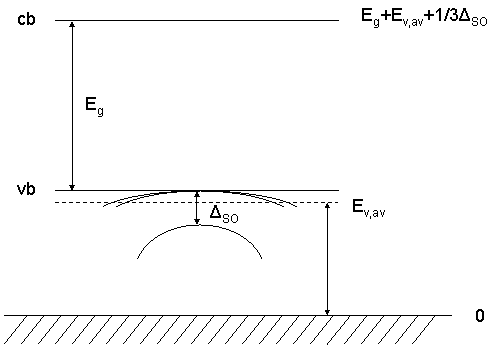The conduction band energies are given for 0 Kelvin in the nextnano³ database.
Varshni parameters should be used to get the temperature dependent conduction band energies for e.g. 300 K.

With the specifier` band-shift` [eV], you are able to shift the absolute values of valence and conduction band edges simultaneously by a certain amount (default: 0.0 eV).

For zinc blende, the three hole energies Ehh, Elh and Eso (heavy, light and spin-orbit split-off energy) are related as follows:
(Ehh + Elh + Eso)/3 = Ev,av

For wurtzite, the three hole energies EA, EB and EC (heavy, light and crystal-field split-off energy) are related as follows:
(EA + EB + EC)/3 = 2/3 * Deltacr + Ev,av

### Summary

VBO: You can adjust` valence-band-energies `and/or``` band-shift ```to adjust the valence band offset.
CBO: Option a) You can adjust` conduction-band-energies `(if``` use-band-gaps = no```) and/or` band-shift ` to adjust the conduction band offset.
Option b) You can adjust``` band-gaps ```(if` use-band-gaps = yes`) and/or` band-shift `and/or` valence-band-energies `adjust the conduction band offset.

### Ternaries

The situation for ternaries is essentially the same.
Bowing parameters can be used to tune the band gaps and band offsets. Additionally, a` band-shift `can be used.
(`bow-conduction-band-energies`,` bow-valence-band-energies`,``` bow-band-shift```,` band-shift`)
For ternaries, the Varshni parameters are not interpolated. Instead, the``` conduction-band-energies ```(if``` use-band-gaps = no```) are interpolated (default) or the band-gaps (if``` use-band-gaps = yes```).

In order to get the valence band edges for ternaries, the following procedure applies.

1. The following parameters are interpolated (including taking into account bowing parameters):` [eV]`
` - `average valence band edge energy Ev,av:``` valence-band-energies = ... [eV] - ```split-off energy Deltaso:``` 6x6kp-parameters = . . . DeltaSO [eV] - ```absolute deformation potential:``` absolute-deformation-potential-vb = ... [eV] - ```uniaxial deformation potentials:``` uniax-vb-deformation-potentials   = ... [eV] - ```band-shift:``` band-shift = ... [eV] ```(Typically, the default value is zero.)
2. The 6-band k.p Hamiltonian is set up including strain tensor.
It is diagonalized and the 6 eigenenergies are called heavy hole, light hole and split-off hole (each of them is two-fold degenerate).
3. Note that the band gap is defined with respect to the unstrained valence band edge maximum. The program takes shifts and splittings of the conduction band edges due to strain automatically into account, i.e. changes of the band gap due to strain are included automatically.

## Database - Conduction/Valence band nonparabolicities

### Question

How is nonparabolicity treated?

### Answer

The following parameters of the database refer to a hyperbolic dispersion,` k2 ~ E(1+aE) `where``` a = ```nonparabolicity` [1/eV]`.```  conduction-band-nonparabolicities = 0d0 0d0 0d0 !  valence-band-nonparabolicities    = 0d0 0d0 0d0 !```

They are only used in regions where the density is calculated classically.
The do not enter the Schrödinger equation.
In order to treat nonparabolicty in quantum regions, a 6-band k.p or 8-band k.p model is required.

A common problem is that for the smaller band gap semiconductors like InAs, the nonparabolicity is so large that the calculated Fermi levels (to be precise: the calculated alignment of the band edges with respect to the Fermi level) are quite inaccurate.
For example, when looking at a case with n~1.5x1018 cm-3, the Fermi level calculated without nonparabolicity is about 0.214 eV. It is 0.143 eV when nonparabolicty is taken into account. Here is an example of how one can modify the Fermi-Dirac integral for free carrier concentration in 3D for a single band from parabolic to nonparabolic using the Van Halen-Pulfrey functions. The nonparabolicity modifies the SQRT(E) density of states in the simple way shown.

## Database - Effective masses

### Question

How are effective hole masses determined in the database? By k.p parameters (`6x6/8x8kp-parameters`) or by effective masses (`valence-band-masses`)?

### Answer

In the database when we describe material we specify

• k.p parameters and
• hole effective masses.

But they are not consistent: k.p parameters are used only when 6-band or 8-band k.p quantum model is selected and hole effective masses are used only when 1-band Schrödinger equation is solved.
(Exception: The k.p parameter Deltaso for spin-orbit splitting energy is used in any case including if no strain is considered. The k.p parameters in the database are also used to determine band shifts due to strain. Program computes the k.p and strain dependent Hamiltonian for each grid point, diagonalizes it, and takes the eigenvalues for the band edges. So, band edges for single band calculations are k.p parameter dependent. More information ...)

There is also the option under``` \$numeric-control ```to calculate the effective valence band masses from the 6-band k.p parameters (L, M, N) specified in the database.

## Dirichlet/Neumann boundary conditions

Question

For the quantum calculation there is a possibility to choose either``` Dirichlet ```or` Neumann `boundary conditions. Which function is a subject for such boundary conditions? Wave function? If yes, do``` Dirichlet ```conditions mean that wave function is equal to zero on the boundaries?

Answer

Yes, at the boundaries of the quantum region, the wave function is zero if``` Dirichlet ```is chosen.
`Neumann`:
- Physically, it means zero current normal to the boundary surface (3D), boundary line (2D) or boundary point (1D).
- Mathematically, it means if a Hamiltonian contains a term like
[d/dxi mij d/dxj + bi d/dxi + c] Psi
then the boundary condition is
[ni mij d/dxj + bi ni] Psi = 0
where ni is a normal vector to a surface (line).
More details about boundary conditions can be found here: Boundary conditions

## Doping concentration

Question 1

In 1D, what is the unit of doping concentration? If I have an experimental value of 2*1018 cm-3 for 3D, is it then 21/3*106 cm-1 for 1D?

Answer 1

No, it is still 2*1018 cm-3 for 1D. Please look at this example for more details: `\$doping-function`

## Errors

Error message` Error linesearch: Round-off problem.`

Solution

This error occurs in the Newton routine (subroutine linesearch) when solving the 1D/2D/3D nonlinear Poisson equation with the Newton method.

Possible solutions (depending on your input file geometry):

• ``` \$numeric-control  ...  nonlinear-poisson-stepmax    = 1.0d-0  nonlinear-poisson-iterations = 100 \$end_numeric-control```
• Try to set different starting values for the initial guess of the potential``` phiV ```under the keyword``` \$numeric-control ```(specifier` initial-potential`).

Error message (on Windows only)
```forrtl: error (74): floating underflow forrtl: error (74): floating underflow forrtl: info (300): 9 floating underflow traps```

Explanation

An underflow indicates that the absolute value of a floating point number has become smaller than about 1e-150 and that it has been replaced by a zero. For many algorithms this replacement does not cause any harm, for others the effects will be disastrous. Unfortunately, in simulation codes like nextnano3 both cases occur depending on where in the code the underflow happened.

## Fermi level

Question

I want to manually adjust the Fermi level. How can I do this?

Answer

There are several possibilites.

• Read in Fermi levels from an ASCII file.
``` \$import-data-on-material-grid```
You can use different Fermi levels for electrons and holes.
• Assign a Fermi level to a region cluster.
`\$region-cluster`
You can use different Fermi levels for electrons and holes.
• Assign a Fermi level to a quantum cluster.
``` \$quantum-cluster```
You can use different Fermi levels for electrons and holes.
• Use "Poisson boundary condition" to assign to a certain region a "Fermi level boundary condition". You can choose a constant Fermi level (`Fermi`) or a linear Fermi level profile (`Fermi-linear`).
``` \$poisson-boundary-conditions```
The same Fermi level is applied to both electron and hole Fermi levels.

These four options can also be combined.

An example is shown here: Double quantum well heterostructure

```1DAlGaAs_GaAs_DQW_read_in_Fermi_level.in 1DAlGaAs_GaAs_DQW_apply_Fermi_level_to_region_cluster.in 1DAlGaAs_GaAs_DQW_apply_Fermi_level_to_quantum_cluster.in 1DAlGaAs_GaAs_DQW_apply_Fermi_level_to_contact.in```

If you are interested in examples, please contact``` support [at] nextnano.com```.

## Growth direction

Question

I have to simulate a quantum dot with `[N 1 1]` growth direction. But in the database I have only:

```axes-possible = 0 0 1 !                 0 1 0 !                 1 0 0 !```

Is it possible to simulate [N 1 1] growth direction?

Answer

Yes, but don't confuse the `axes-possible `specifier with the Miller indices. Change these to `[N 1 1] `for other directions. The specifier` axes-possible `is only applicable to the growth direction which must be x, y or z as indicated. However, you can choose ```[3 1 1] ```to be the Miller x axis and then choose x axis as growth direction which will then be `[3 1 1]`.
More details can be found under keyword ``` \$domain-coordinates```.

## Materials

Question

What should I pay attention to?

Answer

• You can define new materials for certain temperatures that consider a change in the band gap due to temperature (e.g. `GaAs-4K` in addition to `GaAs` in the database).
• AlxGa1-xAs
You should adjust the bowing factor manually:
```  bow-conduction-band-energies  =  0.305d0  0.055d0  0d0 ```The bowing factor for the Gamma band (according to Vurgaftman is)
-0.127+1.310x   for Egap(Gamma)
e.g.   `0.384d0` for Al0.39Ga0.61As```    ->```  Crossover for minimum (Gamma `->` L) at x=0.39   (300 K)
`    0.305d0` for Al0.33Ga0.67As```    -> ```(Gamma)
`    0.070d0` for Al0.15Ga0.85As```    -> ```(Gamma)

## Matlab

Question

Can I use Matlab to visualize the 3D output files (AVS format)?

Answer

Not directly, but you should be able to convert the AVS data to Matlab format if you write a script that does it for you. For an explanation of the AVS format, please consult the 2D/3D Tutorials.

## Piezoelectric charges

Question

I want to "switch-off" piezoelectrical effects. Should I put piezo-electric-constant e14  in the `database_nn3.in` file to be 0, or  can I specify it somewhere in the input file?

Answer

Option 1: You have to adjust e14 for the appropriate materials in the `database_nn3.in` file to:
``` piezo-electric-constants = 0d0  0d0 0d0 0d0 ! [C/m²] e14,B114,B124,B156```

Option 2: You can specify it here:
```  \$numeric-control```
`   piezo-constants-zero = no`

The pyroelectric constants can be switched on and off similarly.

## Quaternaries

Question

Are quaternaries included? If not, how can I interpolate them?

Answer

Only lattice matched-quaternaries (e.g. InxGayAl1-x-yAs with only one free parameter x or y but not quaternaries with two free parameters like AxB1-xCyD1-y) are included in our database. See database comments and quaternaries documentation.

You can define a new material in the``` database_nn3.in ```file with linearly interpolated values of the binary constituents of the quaternary.

## Strain (band shifts and deformation potentials)

Question

How do you incorporate strain into the calculation? What is the meaning of the deformation potentials in the database?

Answer

In order to get the band edge shifts due to strain, we diagonalize numerically on each grid point the strain dependent k.p Hamiltonian for k=0. More information ...

Van de Walle (PRB 39, 1871 (1989)) describes the dependence on strain of the valence and conduction band edges (zinc blende). In this paper strain is described along  and  directions. In a general case, when we have [a b c] growth direction please have a look here.

Pseudomorphic (or commensurate) growth of strained layers on a substrate subjects these layers to a biaxial strain (epsilon||) parallel to the plane of the interface and a uniaxial strain (epsilon|_) perpendicular to it.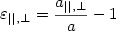(1)

where    a||=a0                                      (2a)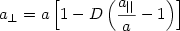.      (2b)

So we can also write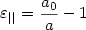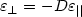Here a0 denotes the lattice constant of the substrate and a the equilibrium lattice constant of the layer material. D depends on the elastic constants c of the layer material and on the interface orientation. (Often, 1/D001 is called the Poisson ratio, denoted as sigma).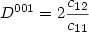(3a)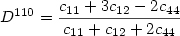(3b)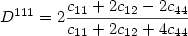(3c)

In the database we specify:

 for zinc blende for wurtzite units `lattice-constants` a     a     a a      a      c nm `elastic-constants` c11  c12   c44 c11  c12  c13  c33   c44 GPa

The lattice constants are temperature dependent. More information...

The effect of strain on energy levels can be decomposed into hydrostatic and shear contributions. The hydrostatic strain component leads to a shift of the average valence band energy Ev,av=(Ehh+Elh+Eso)/3, i.e. of the average of the energies of the heavy hole, light hole and spin-orbit split-off bands (see picture for details):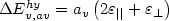(4a)

and similarly for the conduction band energy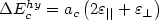(4b),

where av and ac are the hydrostatic (=absolute) deformation potentials for the valence band and conduction band, respectively.

In the database we specify:

 valence bands conduction bands Gamma L X `absolute-deformation-potential-vb` av `absolute-deformation-potentials-cbs` ac(Gamma) ac(L) ac(X)

The shear contribution couples to the spin-orbit interaction and leads to an additional splitting of the valence band energies. In the case of growth on a (001) or (111) substrate, the energy shifts relative to Ehh=Ev,av+Deltaso/3  (Deltaso is the spin-orbit splitting in the absence of strain) are given by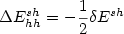(5a)(5b)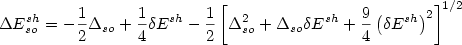(5c)

The strain dependent shift delta Esh depends on the interface orientation: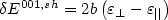(6a)        or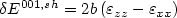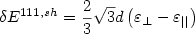(6b)        or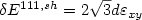Here,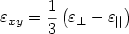.

The quantities b and d are the tetragonal and rhombohedral shear deformation potentials, respectively. In the absence of strain, equation (5) leads to the correct spin-orbit splitting Deltaso. Conduction bands at Gamma are not affected by the shear contribution to the strain. However, at L and X they are affected.

In the database we specify:

 valence bands conduction bands Gamma L X `uniax-vb-deformation-potentials` b          d `uniax-cb-deformation-potentials` 0 (always zero) Xiu(L) Xiu(X) `valence-band-energies` Ev,av    (eV) `conduction-band-energies` Ev,av+   1/3 DeltaSO+   Egap(Gamma) Ev,av+   1/3 DeltaSO+   Egap(L) Ev,av+   1/3 DeltaSO+   Egap(X) `6x6kp-parameters` .....     .....    ..... Deltaso  (eV) `8x8kp-parameters` .....     .....    ..... .....     ....     .....

Once Ev,av are known on an absolute scale (only physically meaningful relative to Ev,av in related semiconductors), the valence band and conduction band edge energies Ev and Ec, respectively, are obtained on an absolute scale from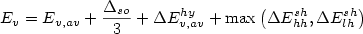(7a)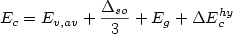(7b)   (for Gamma)

where Ev,av, Deltaso, and Eg (band gap energy) refer to unstrained bulk properties. The strain contribution is incorporated into the other properties.
Now we can calculate the band offset at the interface directly comparing the values of Ev and and Ec of the semiconductors constituting the heterojunction.

Equations (4), (5) and (6) were arrived at by treating the effect of strain as a small perturbation, and are therefore only valid in the case of small lattice mismatch. The mismatch attainable in pseudomorphic heterojunctions composed of III-V alloys is expected to fall within this range of validity.

(Most of this text was taken from M.P.C.M. Krijn, Semicond. Sci. Technol. 6, 27 (1991))

More information ...

## Strain (pseudomorphic and relaxed in one structure simultaneously)

Question

Is it possible to have some of the layers of the heterostructure to be pseudomorphic and then at some point higher up in the structure have the layers be relaxed? Currently, I have the entire structure with``` homogeneous-strain```.

Answer

Yes, it is. Have a look at this keyword:``` \$material```
For each material one can specify its own substrate. If this substrate is identical to the material name, then one has a "relaxed" and not a strained material, even with option` homogeneous-strain`.

## Substrate

Question

How can I specify the substrate or its lattice constant?

Answer

``` \$domain-coordinates  ...  pseudomorphic-on = ```<material-name>
``` ... \$end_domain-coordinates```

## Superlattice

Question

I want to simulate a superlattice of quantum wells in 1D. For this I want to build a cluster consisting of a well and a barrier, i.e. this cluster would contain 2 different materials. Is this possible?

Answer

No. You can define one huge cluster that consists of the barrier material for the whole superlattice and set` region-priority=1`. Then you can put several wells on it with` region-priority=2`. Alternatively, open two windows of the 1D Input file generator (Step 1) and define in the first the barrier and in the second the well and press the "Submit" buttons alternately.

## Temperature

Question

What physical properties or equations are affected if I change the``` lattice-temperature ``` in the input file?

Answer

• Band gap?
Yes! The latest version includes temperature dependent band gaps based on the Varshni parameters that are specified in the database. (Actually only the conduction band energy is changed. The valence band energy remains constant.)
You can switch it off:``` varshni-parameters-on = no```.
No! (Previous versions) The band gap is specified by values on an absolute scale which can be changed manually in the``` database_nn3.in ```file to get the correct band gap for the corresponding temperature of interest (more information on band gap). You can even specify your own materials as was already done for GaAs-4K, AlAs-4K, InAs-4K, Al(x)Ga(1-x)As-4K, In(x)Ga(1-x)As-4K, Al(x)In(1-x)As-4K (in addition to GaAs, AlAs, ...).
• Doping?
Yes! To what degree are the donors or acceptors ionized or not? This is clearly a function of temperature. The keyword``` \$impurity-parameters ``` specifies how far a donor/acceptor lies below/above the conduction/valence band. If the temperature is around 4 K, almost no donors/acceptors are ionized. In this case - if you still want all donors/acceptors to be fully ionized - you need to specify a value of say -10 eV instead of +0.03 eV.
• Mobility?
Yes!
• Lattice constants?
Yes!
You can switch in on or off under keyowrd``` \$numeric-control (lattice-constants-temp-coeff-on = no/yes```).``` ```No! (Previous versions)

Question

Can I change the``` lattice-temperature ``` to 0 Kelvin in the input file?

Answer

```-> \$program-restrictions```

## Orientation in 2D (simulation system)

Question

When we have 2D simulation we can specify as simulation domain``` [1 0 1] ```or` [1 1 0 ] `or` [0 1 1]`. What is` x ` and what is` y `in the simulation domain?

Answer

 [x y z] simulation system calculation sytem = x,y system [1 0 1] (x,z)-plane x=x, y=z [1 1 0] (x,y)-plane x=x, y=y [0 1 1] (y,z)-plane x=y, y=z

## Output files

Question 1

How to plot 2D AVS/Express output data with Origin?```  ->  ``` Answer

## Units - electron density, elastic constants

Question 1

What is the unit of the electron density (output)? What is the units of the elastic constants in the database?

Answer 1

The electron/hole density output will be given in the following units `[````1018 cm-3````]`. These units are the same for 1D, 2D and 3D simulations.
The elastic constants are given in GPa.

Question 2

The numbers of the electron density on my plot looks different to what I expected.

Answer 2

You could try to adjust your` energy-levels-relative `in``` impurity-parameters ```to` -10d0 `instead of e.g. `0.02d0` and check if it makes a difference.

Question 3

I get three different columns for my electron density output (1D) when I run a quantum mechanical calculation. Which one is the electron density?

Answer 3

The second column in the electron density which is the sum of classical and quantum mechanical density.
- second column: sum of quantum mechanical and classical density
- third column:     quantum mechanical density
- forth column:     classical density

## Warning: ```Warning define_poisson_clusters: Boundary conditions fixed manually, not by input.```

Question

I get the following warning:
```Warning define_poisson_clusters: Boundary conditions fixed manually, not by input. ```What does it mean?

Answer

It simply means that for the boundary conditions of the Poisson equation (`\$poisson-boundary-conditions`) by default Neumann boundary conditions with electric field = 0 V/m are assumed when calling` SUBROUTINE define_poisson_clusters`. This is just a reminder that these boundary conditions cannot be specified in the input file yet. However, `Ohmic `and``` Schottky ```boundary conditions are implemeted correctly.

## Calculating eigenvalues for 8-band k.p

Question

Why does it take so long to calculate the eigenvalues of an 8-band k.p calculation?

Answer

Mathematically speaking, 8-band k.p requires a different eigenvalue solver (“finding eigenvalues in the interior of the spectrum”) than single-band and 6-band (“finding eigenvalues at the end of the spectrum”), so it is much harder (both with respect to time and stability) to find a solution.

An 8-band matrix has 8 times the size of a single-band matrix and the mathematical problem gets harder the more eigenvalues are sought.

We have implemented different eigenvalue solvers for 8-band k.p, e.g. the ARPACK solver (shift-invert mode) or the FEAST solver.

Degeneracy: For instance, if 4 eigenvalues are degenerate (e.g. due to spin and/or geometry) and you are looking for only 3, the eigenvalue solver will very likely fail. In such a case, it could be that certain eigenvalues are misisng.

Adjusting the resdiual might help you for debugging, e.g. by using a larger value for the residual (accuracy) one should get solutions more quickly althouth they might not be accurate enough.

Another interesting strategy could be to divide the input file into two, e.g. if one needs 6 electron eigenvalues and 12 hole eigenvalues.
- One input file calculates only the electron eigenvalues. Then you have in total 6 eigenvalues instead of 18 which should be much faster.
- The other input file calculates only the hole eigenvalues. (12 instead of 18)